Courses

# Advanced Topics - Metal Oxide Semiconductor Field Effect Transistor (MOSFET) Electrical Engineering (EE) Notes | EduRev

## VLSI System Design

Created by: Machine Experts

## Electrical Engineering (EE) : Advanced Topics - Metal Oxide Semiconductor Field Effect Transistor (MOSFET) Electrical Engineering (EE) Notes | EduRev

The document Advanced Topics - Metal Oxide Semiconductor Field Effect Transistor (MOSFET) Electrical Engineering (EE) Notes | EduRev is a part of the Electrical Engineering (EE) Course VLSI System Design.
All you need of Electrical Engineering (EE) at this link: Electrical Engineering (EE)

Objectives

In this course you will learn the following

• Motivation for Scaling
• Types of Scaling
• Short channel Effect
• Velocity saturation

7.1 Motivation for Scaling

The reduction of the dimensions of a MOSFET has been dramatic during the last three decades. Starting at a minimum feature length of 10 mm in 1970 the gate length was gradually reduced to 0.15 mm minimum feature size in 2000, resulting in a 13% reduction per year. Proper scaling of MOSFET however requires not only a size reduction of the gate length and width but also requires a reduction of all other dimensions including the gate/source and gate/drain alignment, the oxide thickness and the depletion layer widths. Scaling of the depletion layer widths also implies scaling of the substrate doping density.

In short, we will study simplified guidelines for shrinking device dimensions to increase transistor density & operating frequency and reduction in power dissipation & gate delays.

7.2 Types of Scaling

Two types of scaling are common:

(i) constant field scaling and

(ii) constant voltage scaling

Constant field scaling yields the largest reduction in the power-delay product of a single transistor. However, it requires a reduction in the power supply voltage as one decreases the minimum feature size.

Constant voltage scaling does not have this problem and is therefore the preferred scaling method since it provides voltage compatibility with older circuit technologies. The disadvantage of constant voltage scaling is that the electric field increases as the minimum feature length is reduced. This leads to velocity saturation, mobility degradation, increased leakage currents and lower breakdown voltages.

After scaling, the different Mosfet parameters will be converted as given by table below :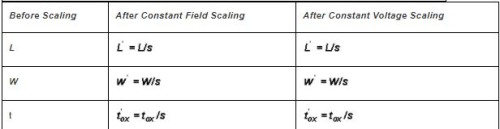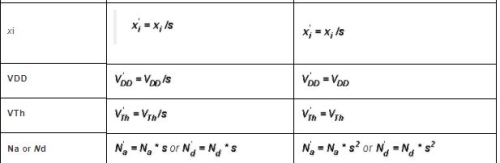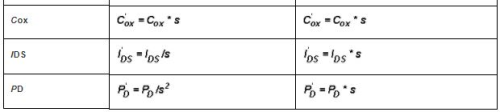Where s=scaling parameter of MOS

7.3 Short Channel Effect

So far our discussion was based upon the assumptions that channel was long and wide enough, so that “edge” effects along the four sides was negligible, longitudinal field was negligible and electric field at every point was perpendicular to the surface. So we could perform one-dimensional analysis using gradual channel approximation. But in devices where channel is short longitudinal field will not be negligible compared to perpendicular field. So in that case one-dimensional analysis gives wrong results and we will have to perform dimensional analysis taking into account both longitudinal and vertical fields. (which is out of the scope this course)

When is a channel called a short channel?
(i) When junction (source/drain) length is of the order of channel length.
(ii) L is not much larger then the sum of the drain and source depletion width.

We have shown below the comparative graphs of   I-V characteristics for both long channel and short channel length MOSFETs. From graph, it can be clearly concluded that when the channel becomes short, the current in saturation region becomes linearly dependent on applied drain voltage rather than being square dependent.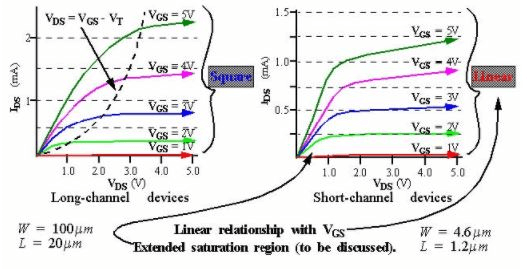Figure 7.3: Comparison of ID vs VDS characteristics for long and short channel MOSFET devices

7.4 Velocity Saturation

As we were assuming longitudinal field to be very small in the channel, the magnitude of carrier velocity |vd| was proportional to |Ex|. But it has been observed that for high values of |Ex| carrier velocity tends to saturate. It is no more proportional to |Ex|. This lack of proportionality at high |Ex| values is known as velocity saturation.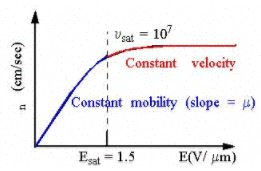Figure 7.4 Velocity Saturation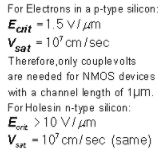Offer running on EduRev: Apply code STAYHOME200 to get INR 200 off on our premium plan EduRev Infinity!

,

,

,

,

,

,

,

,

,

,

,

,

,

,

,

,

,

,

,

,

,

;